## Example Questions

← Previous 1 3 4 5

### Example Question #1 : How To Find Consecutive Integers

The sum of three consecutive even integers is 108.  What is the largest number?

38

40

36

42

34

38

Explanation:

Three consecutive even integers can be represented by x, x+2, x+4.  The sum is 3x+6, which is equal to 108.  Thus, 3x+6=108.  Solving for x yields x=34.  However, the question asks for the largest number, which is x+4 or 38.  Please make sure to answer what the question asks for!

You could have also plugged in the answer choices.  If you plugged in 38 as the largest number, then the previous even integer would be 36 and the next previous even integer 34.  The sum of 34, 36, and 38 yields 108.

### Example Question #2 : How To Find Consecutive Integers

The sum of three consecutive even integers equals 72. What is the product of these integers?

17472

13800

12144

13728

10560

13728

Explanation:

Let us call x the smallest integer. Because the next two numbers are consecutive even integers, we can call represent them as x + 2 and x + 4. We are told the sum of x, x+2, and x+4 is equal to 72.

x + (x + 2) + (x + 4) = 72

3x + 6 = 72

3x = 66

x = 22.

This means that the integers are 22, 24, and 26. The question asks us for the product of these numbers, which is 22(24)(26) = 13728.

### Example Question #3 : How To Find Consecutive Integers

Four consecutive integers have a mean of 9.5. What is the largest of these integers?

11

9

13

12

8

11

Explanation:

Four consecutive integers could be represented as n, n+1, n+2, n+3

Therefore, by saying that they have a mean of 9.5, we mean to say:

(n + n+1 + n+2 + n+ 3)/4 = 9.5

(4n + 6)/4 = 9.5 → 4n + 6 = 38 → 4n = 32 → n = 8

Therefore, the largest value is n + 3, or 11.

### Example Question #4 : How To Find Consecutive Integers

The sum of four consecutive odd integers is equal to 96. How many of the integers are prime?

1

2

3

4

0

1

Explanation:

Let x be the smallest of the four integers. We are told that the integers are consecutive odd integers. Because odd integers are separated by two, each consecutive odd integer is two larger than the one before it. Thus, we can let + 2 represent the second integer, + 4 represent the third, and + 6 represent the fourth. The sum of the four integers equals 96, so we can write the following equation:

x + (+ 2) + (+ 4) + (+ 6) = 96

Combine x terms.

4x + 2 + 4 + 6 = 96

Combine constants on the left side.

4x + 12 = 96

Subtract 12 from both sides.

4x = 84

Divide both sides by 4.

x = 21

This means the smallest integer is 21. The other integers are therefore 23, 25, and 27.

The question asks us how many of the four integers are prime. A prime number is divisible only by itself and one. Among the four integers, only 23 is prime. The number 21 is divisible by 3 and 7; the number 25 is divisible by 5; and 27 is divisible by 3 and 9. Thus, 23 is the only number from the integers that is prime. There is only one prime integer.

### Example Question #5 : How To Find Consecutive Integers

The sum of three consecutive integers is 60. Find the smallest of these three integers.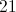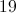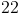Explanation:

Assume the three consecutive integers equal,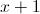, and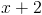. The sum of these three integers is 60. Thus,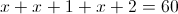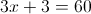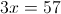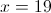### Example Question #6 : How To Find Consecutive Integers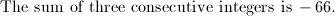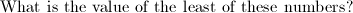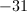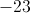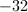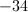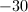Explanation: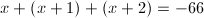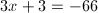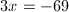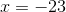### Example Question #7 : How To Find Consecutive Integers

In the repeating pattern 9,5,6,2,1,9,5,6,2,1......What is the 457th number in the sequence?

1

9

2

1

5

5

Explanation:

There are 5 numbers in the sequnce.

How many numbers are left over if you divide 5 into 457?

There would be 2 numbers!

The second number in the sequence is 9,5,6,2,1

### Example Question #8 : How To Find Consecutive Integers

Ifare consecutive, non-negative integers, how many different values of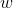are there such that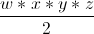is a prime number?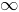Explanation:

Since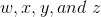are consecutive integers, we know that at least 2 of them will be even. Since we have 2 that are going to be even, we know that when we divide the product by 2 we will still have an even number.  Since 2 is the only prime that is even, we must have: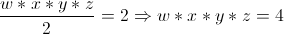What we notice, however, is that for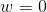, we have the product is 0.  For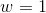, we have the product is 24.  We will then never have a product of 4, meaning thatis never going to be a prime number.

### Example Question #9 : How To Find Consecutive Integers

Four consecutive odd integers have a sum of 32. What are the integers?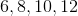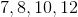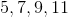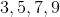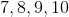Explanation:

Consecutive odd integers can be represented as x, x+2, x+4, and x+6.

We know that the sum of these integers is 32. We can add the terms together and set it equal to 32:

x + (x+2) + (x+4) + (x+6) = 32

4x + 12 = 32

4x = 20

x = 5; x+2=7; x+4 = 9; x+6 = 11

Our integers are 5, 7, 9, and 11.

### Example Question #1 : Sequences

How many integers in the following infinite series are positive: 100, 91, 82, 73 . . . ?

9

12

13

11

10